# Quiz 13: General Equilibrium and Welfare

The production possibility frontier shows different combinations of two goods which can be produces with given technology and resources. a. The production possibility frontier for guns (x) and butter (y) is given below: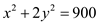This is an equation of a parabola. The production possibility frontier for the guns and butter given below: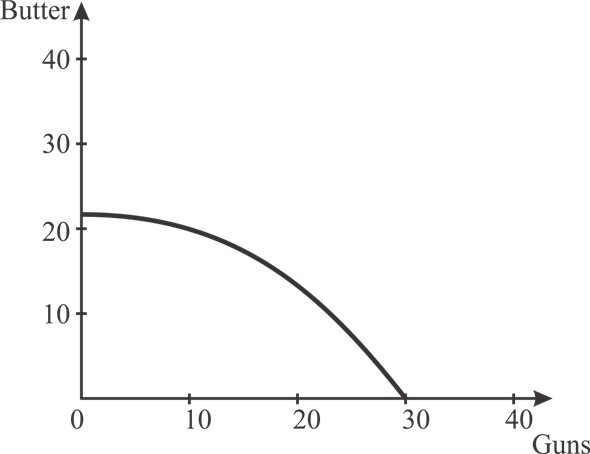Figure 1 On X axis the units of guns is shown and on Y axis the units of butter is shown. PPF curve shows the combination of guns and butter that can be produced with in given resources. b. If individuals always choose consumption bundles in which y = 2x so the production should be in this ratio only. Put value of y in the production possibility equation as shown below:Put value of x in the equation of y as shown below: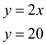If individuals always prefer consumption bundles in which y = 2x, then 10 units of x and 20 units of y will be produced. The rate of product transformation (RPT) between two outputs is the negative of the slope of the production possibility frontier of those outputs. The formula for the RPT is given below: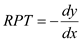The production possibility frontier is given below: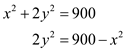Differentiate both sides with respect to x as shown below:RPT is calculated at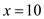and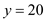as shown below: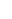At a point where x = 10 and y = 20, the rate of product transformation (RPT) is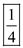. At optimality the price ration is same as RPT Hence, the price ratio that will cause production to take place at this point is. d. The solution point is shown in the figure below by the point A.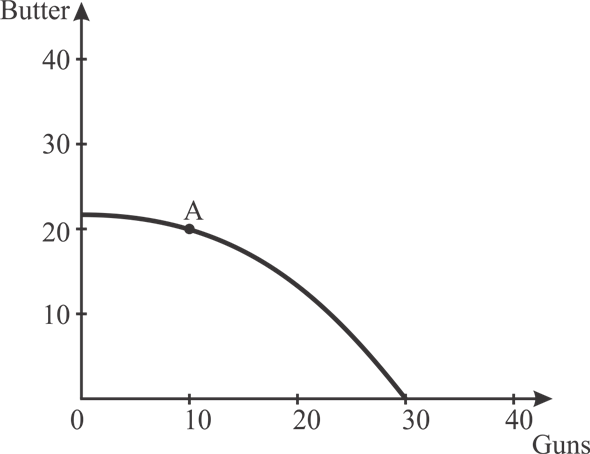Figure 2 On X axis the units of guns is shown and on Y axis the units of butter is shown. PPF curve shows the combination of guns and butter that can be produced with in given resources. Point A shows the combination where, x = 10 and y = 20.
A utility function represents individual's preferences for consuming bundle of goods and services, it calculates individual's preferences in numerical terms. Utility function assign a numerical value to a particular bundle according to its preferences.  As per the question, Person S and J each have 10 hours of labour for the production of ice-cream or chicken. The quantity of ice-cream and chicken is represented by x and y respectively. The utility function of Person S is: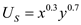The utility function of Person J is: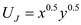The production function of ice-cream and chicken are: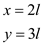where, l is labor employed a. In order to calculate the price ratio,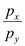, first profit is maximized for both ice-cream and chicken. The profit of ice-cream is maximized. The value of x is put in the given situation as shown below: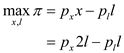In this the total revenue earned from ice-cream is subtracted from total cost incurred. Now, it is differentiated with respect to labor, to maximize the profit.The value of p x is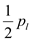. …… (1) The profit of chicken is maximized. The value of y is put in the given situation as shown below: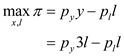In this the total revenue earned from chicken is subtracted from total cost incurred. Now, it is differentiated with respect to labor, to maximize the profit.The value of p y is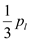. …… (2) Now, the price ratio is calculated by using values from (1) and (2) as shown below:Dividing these two equations, the following is derived: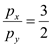Thus, the price ratio is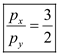. b. First, the quantity demanded by Person S is calculated. In order to calculate the quantity demanded of x and y , the utility function of Person S is maximized subject to his budget constraint. The total number of labor hours is 10. The values of price of ice-cream and chicken are taken from (1) and (2). The budget constraint of Person S is shown below:In the above equation, x 1 is ice-cream demanded by Person S y 1 is chicken demanded by Person S The condition of utility maximization is shown below: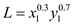Subject to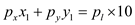. Now, using Lagrangian, the following is obtained as shown below: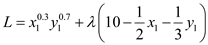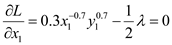…… (3)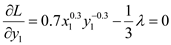…… (4)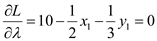Now, dividing equations (3) and (4), the following is derived:Therefore, the value of y 1 is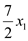. …… (5) The value obtained of y 1 in (5) is substituted in the budget constraint in following manner as shown:The value of y 1 is calculated as shown below:Thus, for Person S x 1 is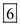and y 1 is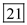. Similarly, the quantity demanded for Person J is calculated. The budget constraint for Person J is shown below:In the above equation, x 1 is ice-cream demanded by Person J y 1 is chicken demanded by Person J The condition of utility maximization is shown below: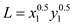Subject to. Now, using Lagrangian, the following is obtained as shown below: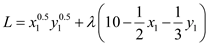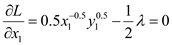…… (6)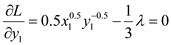…… (7)Now, dividing equations (6) and (7), the following is derived:Therefore, the value of y 1 is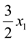. …… (8) The value obtained of y 1 in (8) is substituted in the budget constraint in following manner as shown:The value of y 1 is calculated as shown below:Thus, for Person S x 1 is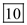and y 1 is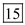. c. It is given that the production function of ice-cream and chicken are:where, l = labor employed To calculate the number of hours spent on the production of ice-cream, first total number of ice-cream produced is calculated. The total number of ice-cream produced is computed as follows:Now, the labor allocated to production of ice-cream is calculated as follows: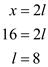Thus, the labor devoted to the production of ice-cream is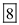. To calculate the number of hours spent on the production of chicken, first total number of chicken produced is calculated. The total number of chicken produced is computed as follows:Now, the labor allocated to production of Chicken is calculated as follows: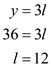Thus, the labor devoted to the production of Chicken is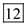.
a) In this case, labor has a limit of 100. The PPF would look like below.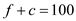The corresponding PPF graph is given below in Figure 1.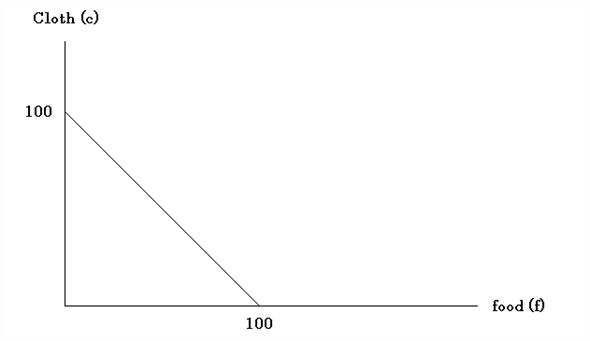Figure 1 b) In this case, land has a limit of 150. The PPF would look like below.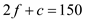The corresponding PPF graph is given below in Figure 2.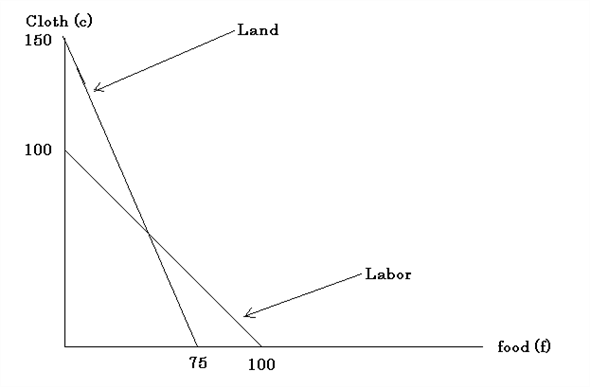Figure 2 c) The combined PPF (ABC) is shown below in Figure 3.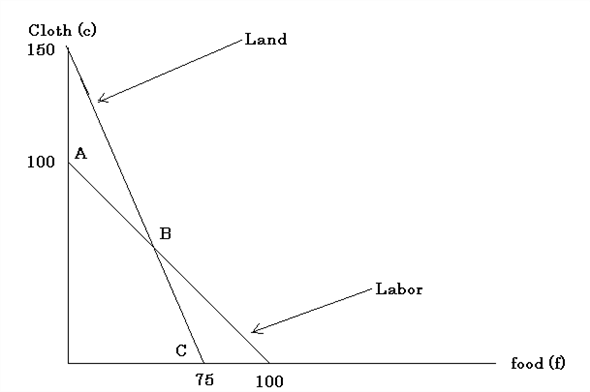Figure 3 d) The combined PPF is concave because of increasing rate of product transformation. In the case of labor, RPT is 1 and in the case of land, RPT is 2. e) The relative price is shown by curve DBE in Figure 4. The slope of curve represents the relative price. Before B, slope is 1 and after B, slope is 2.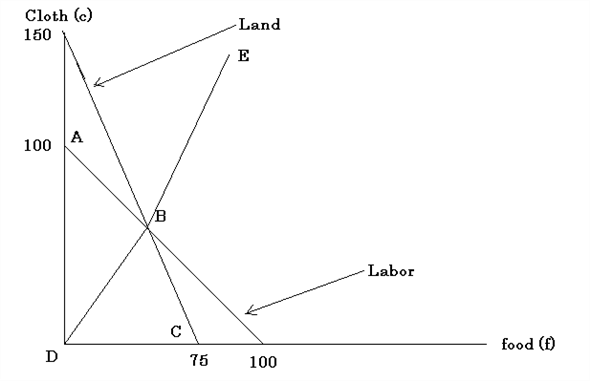Figure 4 f) The individual can buy 4 units of food by paying 5 units of cloth. So, the relative price is 5/4. g) We have seen in Figure 4, the way the relative price changes at point B. The relative price rises instantaneously from 1 to 2. So, the production would remain the same whatever it is at point B. h) The PPF for capital is given in equation below and sketched in Figure 5.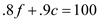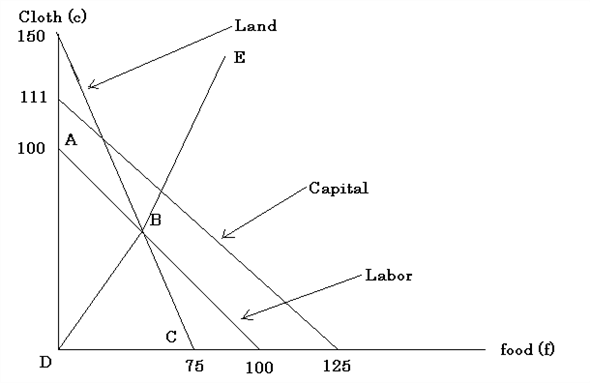Figure 5